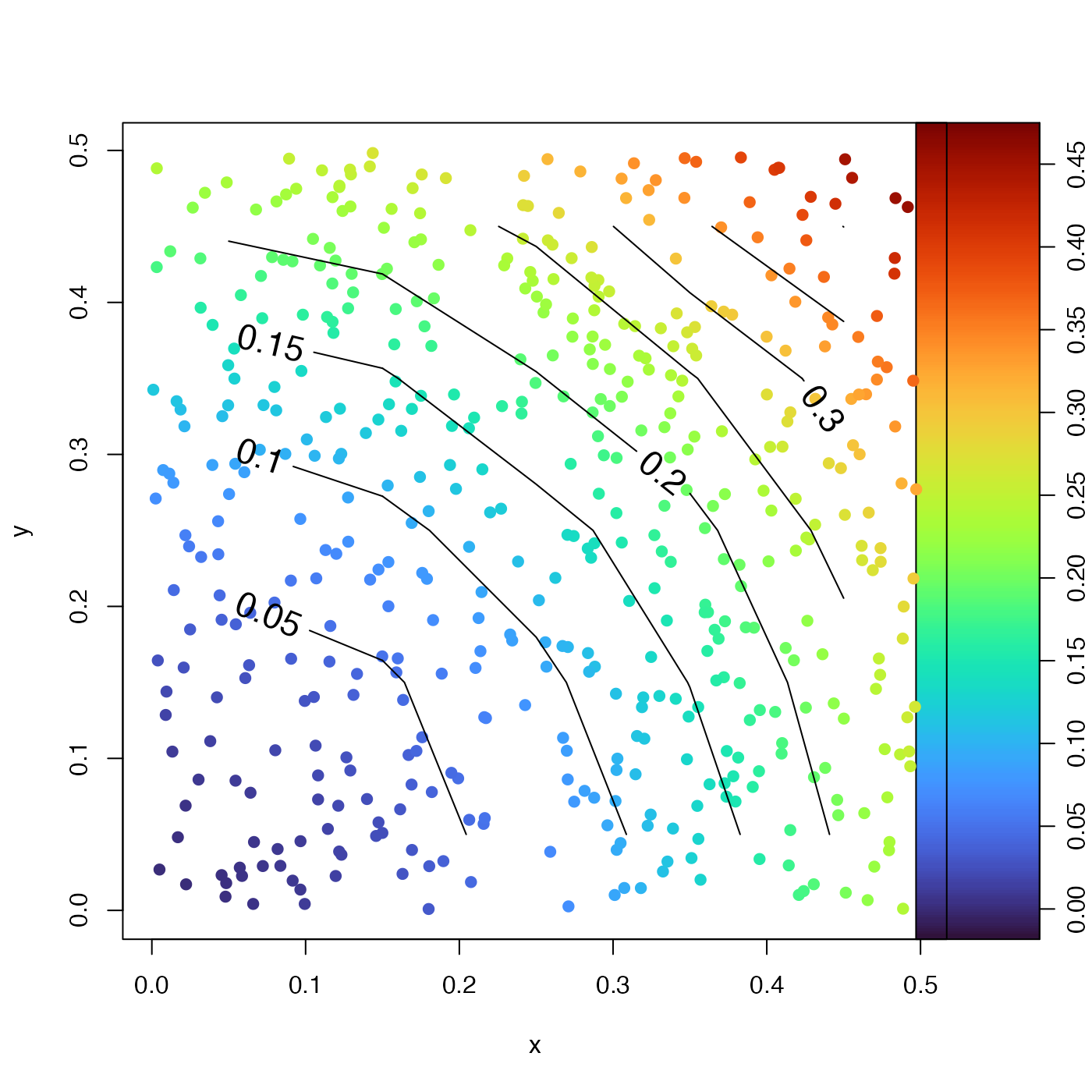Average the values of a vector f(x,y) in bins defined on vectors x and y. A common example might be averaging spatial data into location bins.

binMean2D(
x,
y,
f,
xbreaks,
ybreaks,
flatten = FALSE,
fill = FALSE,
fillgap = -1
)

## Arguments

x vector of numerical values. vector of numerical values. Matrix of numerical values, a matrix f=f(x,y). Vector of values of x at the boundaries between bins, calculated using pretty(x) if not supplied. Vector of values of y at the boundaries between bins, calculated using pretty(y) if not supplied. A logical value indicating whether the return value also contains equilength vectors x, y, z and n, a flattened representation of xmids, ymids, result and number. Logical value indicating whether to fill NA-value gaps in the matrix. Gaps will be filled as the average of linear interpolations across rows and columns. See fillgap, which works together with this. Integer controlling the size of gap that can be filled across. If this is negative (as in the default), gaps will be filled regardless of their size. If it is positive, then gaps exceeding this number of indices will not be filled.

## Value

A list with the following elements: the midpoints (renamed as x and y), the count (number) of f(x,y) values for x and y values that lie between corresponding breaks, and the resultant average (f) of f(x,y), classified by the x and y breaks.

Other bin-related functions: binApply1D(), binApply2D(), binAverage(), binCount1D(), binCount2D(), binMean1D()

## Examples

library(oce)
x <- runif(500)
y <- runif(500)
f <- x + y
xb <- seq(0, 1, 0.1)
yb <- seq(0, 1, 0.2)
m <- binMean2D(x, y, f, xb, yb)
plot(x, y)contour(m$xmids, m$ymids, m\$result, add=TRUE, levels=seq(0, 2, 0.5), labcex=1)